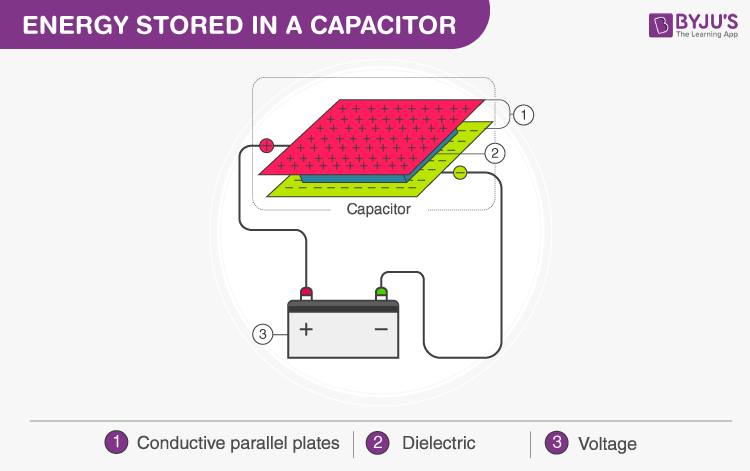# Energy Stored in a Capacitor

The dramatisation that is usually seen in movies where medical personnel uses a defibrillator to pass an electric current through a patient’s heart to get it to beat normally uses the energy stored in a capacitor. Less dramatic application of the energy stored in the capacitor lies in the use of capacitors in microelectronics, such as handheld calculators. In this article, we discuss the energy stored in the capacitor and the formula used to calculate the energy stored in a capacitor.

## How to calculate the energy stored in a capacitor?The energy stored in a capacitor is nothing but the electric potential energy and is related to the voltage and charge on the capacitor. If the capacitance of a conductor is C, then it is initially uncharged and it acquires a potential difference V when connected to a battery. If q is the charge on the plate at that time, then

$$\begin{array}{l}q=CV\end{array}$$

The work done is equal to the product of the potential and charge. Hence, W = Vq

If the battery delivers a small amount of charge dQ at a constant potential V, then the work done is

$$\begin{array}{l}dW=Vdq=\frac{q}{C}dq\end{array}$$

Now, the total work done in delivering a charge of an amount q to the capacitor is given by

$$\begin{array}{l}W=\int_{0}^{q}\frac{q}{C}dq=\frac{1}{C}\frac{q^2}{2}=\frac{1}{2}\frac{q^2}{C}\end{array}$$

Therefore the energy stored in a capacitor is given by

$$\begin{array}{l}U=\frac{1}{2}\frac{q^2}{C}\end{array}$$

Substituting

$$\begin{array}{l}q=CV\end{array}$$
in the equation above, we get

$$\begin{array}{l}U=\frac{1}{2}CV^2\end{array}$$

The energy stored in a capacitor is given by the equation

$$\begin{array}{l}U=\frac{1}{2}CV^2\end{array}$$
.

Let us look at an example, to better understand how to calculate the energy stored in a capacitor.

Example: If the capacitance of a capacitor is 50 F charged to a potential of 100 V, Calculate the energy stored in it.

Solution:

We have a capacitor of capacitance 50 F that is charged to a potential of 100 V. The energy stored in the capacitor can be calculated as follows

$$\begin{array}{l}U=\frac{1}{2}CV^2\end{array}$$

Substituting the values, we get

$$\begin{array}{l}U=\frac{1}{2}50(100)^2=250\times 10^3\,J\end{array}$$

### Applications of Capacitor Energy

Following are a few applications of capacitor energy:

• A defibrillator that is used to correct abnormal heart rhythm delivers a large charge in a short burst to a person’s heart. Applying large shocks of electric current can stop the arrhythmia and allow the body’s natural pacemaker to resume its normal rhythm. A defibrillator uses the energy stored in the capacitor.
• The audio equipment, uninterruptible power supplies, camera flashes, pulsed loads such as magnetic coils and lasers use the energy stored in the capacitors.
• Super capacitors are capable of storing a large amount of energy and can offer new technological possibilities.